# Numerical simulation of turbulent spatial flow of steam layers in exhaust of solar vapor producer using les (Convective Mach number of 0.64)

Numerical simulation of turbulent spatial flow of steam layers in exhaust of solar vapor producer using les (Convective Mach number of 0.64)

Department Mechanical Engineering, Urmia University of Technology, Urmia P.O. Box 57155-419, Iran

Corresponding Author Email:
Page:
33-41
|
DOI:
https://doi.org/10.18280/psees.010105
24 August 2017
| |
Accepted:
29 September 2017
| | Citation

OPEN ACCESS

Abstract:

In this article, the results from three-dimensional numerical simulation of compressible turbulent spatial mixing layers for the vapor flow in a solar producer at Convective Mach number of 0.64, using the Large Eddy Simulation (LES) are presented. The governing equations and LES simulation method are combined to form of a three dimensional computer program on the base of finite volume. The purpose of this research is realizing of LES technique in the compressible turbulent spatial mixing layer in supersonic flows. The dynamic eddy viscosity model is applied for modeling of sub-grid scales. Compressible LES not only require the modeling of the sub-grid terms in the momentum equation, but also the modeling of the sub-grid terms in the energy equation which play the main role at supersonic flows. The present numerical simulation results are compared with experimental and DNS and other LES results in the same convective Mach number. In addition, the rate of total kinetic energy dissipation into heat in sub-grid scales is investigated. The present simulation results are predicted that by increasing convective Mach number, the mixing layers growth rate decreases. Finally, the vorticity during the mixing and shocks occur in the flow field is investigated and discussed in the more details.

Keywords:

LES, compressible flow, spatial mixing layers, sub-grid scales, dynamic eddy viscosity model

1. Introduction

Large Eddy simulation (LES) is an outstanding technique in numerical simulation of turbulent flows. In LES, at first the Navier-Stokes equations should be filtered, in this way it is possible to separate the large and sub-grid scale eddies from each other. After filtering, in addition to filtered terms, the unknown terms are added to the equations. Filtered terms, indicating that the filtered Navier-Stokes equations have been solved using a numerical algorithm and the results of this solution are the filtered variables which show large scales flow fields. The unknown terms are sub-grid scales terms that should be modeled. The modeling of sub-grid scales terms called sub-grid scales modeling. These terms indicate the effect of sub-grid scales flow. The dynamic eddy viscosity model has been formulated by Germano et al. . Based on this model, the amount of flow kinetic energy by eddy viscosity at sub-grid scales is lost to heat. In this model, the square of constant coefficient in Smagorinsky’s  base model has been replaced by the dynamic coefficient, controlling the amount of flow turbulence. This coefficient varies locally to determine the order of eddy viscosity in the flow and subsequently after a time step or several steps by reviewing the flow field, the new value for dynamic coefficient at any point in flow field is achieved again. Thus the value of this coefficient is continually modified. Thus, the correct amount of kinetic energy dissipation in to heat can be produced. The dynamic eddy viscosity model uses Filtering test that its filter width is twice that of the original filter.

Free compressible shear layer in many industrial complex issues is important. One type of shear flows is compressible mixing layers. Mixing layers are examined within the temporal and spatial. Because the temporal frame to show all the features of the growth rate of mixing layer is incapable, framework of spatial mixing layers is used to illustrate characteristics of mixing layers. Spatial mixing layers are made of two parallel flow directions with different speeds. One of the basic parameters of the mixing layers simulation is convective Mach number which has been presented by Bognadoff  that represents the intrinsic compressibility effects in mixing layers. Doris et al.  has been showed the mixing layer growth rate at convective Mach number of 0.64, with LES. Numerical Simulation of spatial mixing layers, by Bruin , at Mach number of 0.2, with LES and DNS has been performed. Large eddy simulation of a droplet laden turbulent mixing layer has been studied by Jones et al. . The experimental data of Goebel & Dutton  have been predicted that with increasing convective Mach number, compressible mixing layer growth rate is reduced. Numerical Simulation of the temporal mixing layers, by Verman , in different convective Mach numbers, has been performed with LES and DNS. He compared sub-grid scales models in his own simulation. Also, Pantano & Sarkar  presented numerical simulation results of temporal mixing layers, using DNS, at different convective Mach numbers. Kourta & Sauvage  simulated supersonic mixing layers with direct numerical simulations to study the flow structures. To study compressibility effects on turbulence, Freud et al.  investigated direct numerical simulations of time evolving of angular mixing layers.

In this article, results from three dimensional numerical simulation of compressible turbulent spatial mixing layers at convective Mach number of 0.64 using LES are presented. The objective of this study is to perform LES of spatially evolving mixing layers and to see if the LES is able to predict the compressibility effects, such as the reduced growth rate and of the dissipated kinetic energy. Finally, Results are compared with experimental data and with the DNS results and other LES results in the same of convective Mach number.

2. Filtering in Les

Filtering is performed due to decompose sub-grid scales from large scales. The arbitrary variable $f$ is filtered by the following spatial integration:

$\overline{f}(x)=\int_{D} f\left(x^{\prime}\right) G\left(x, x^{\prime}\right) d x^{\prime}$    (1)

where, $\overline{f}$ is the filtered part of $f$ which representing the large scales. In Eq. 1, D and G are computational domain and filtering function, respectively. In this study, the top-hat filter is applied which is defined as:

$G(x)=\left\{\begin{array}{ll}{\frac{1}{\Delta}} & {\text { If }|x| \leq \Delta / 2} \\ {0} & {\text { Otherwise }}\end{array}\right.$    （2）

where, Δ is the filtering width, which indicates the resolved scales. The Favre filtering is used in compressible flows, which is suitable for LES. This filtering is denoted by a symbolwhich is related tofiltering by the following relationship:

$\tilde{f}=\frac{\overline{\rho f}}{\overline{\rho}}$    （3）

After the Favre filtering, the $f$ is expressed as:

$f=\tilde{f}+f^{\prime \prime}$    (4)

$\tilde{f}$ and $f^{\prime \prime}$ represent the large and sub-grid scales, respectively. So, the total filtering width is obtained by $\Delta=\left(\Delta_{1} \Delta_{2} \Delta_{3}\right)^{\frac{1}{3}}$.

3. Governing Equations

The governing equations of LES are filtered Navier-Stokes equations can be written as continuity, momentum and energy equations for compressible flows as:

$\partial_{\mathrm{t}} \overline{\rho}+\partial_{\mathrm{j}}\left(\overline{\rho} \tilde{\mathrm{u}}_{\mathrm{j}}\right)=0$    （5）

$\partial_{t}\left(\overline{\rho} \tilde{u}_{i}\right)+\partial_{j}\left(\overline{\rho} \tilde{u}_{i} \tilde{u}_{j}\right)+\partial_{i} \overline{p}-\partial_{j} \check{\sigma}_{i j}=-\partial_{j}\left(\overline{\rho} \tau_{i j}\right)+\partial_{j}\left(\overline{\sigma}_{i j}-\right.\check{\sigma}_{i j} )$    （6）

$\partial_{t} \breve{e}+\partial_{j}\left((\breve{e}+\overline{p}) \tilde{u}_{i}\right)-\partial_{j}\left(\check{\sigma}_{i j} \tilde{u}_{i}\right)+\partial_{j} \check{q}_{j}=-\alpha_{1}-\alpha_{2}-\alpha_{3}+\alpha_{4}+\alpha_{5}+\alpha_{6}$    （7）

$\alpha_{1}=\tilde{u}_{i} \partial_{j}\left(\overline{\rho} \tau_{i j}\right)$    （8）

$\alpha_{2}=\partial_{j}\left(\overline{p u}_{j}-\overline{p} \tilde{u}_{j}\right) /(\gamma-1)$   （9）

$\alpha_{3}=\overline{p \partial_{j} u_{j}}-\overline{p} \partial_{j} \tilde{u}_{j}$    （10）

$\alpha_{4}=\overline{\sigma_{\imath j} \partial_{j} u_{i}}-\overline{\sigma}_{i j} \partial_{j} \tilde{u}_{i}$    （11）

$\alpha_{5}=\partial_{j}\left(\overline{\sigma}_{i j} \tilde{u}_{i}-\check{\sigma}_{i j} \tilde{u}_{i}\right)$    (12)

$\alpha_{6}=\partial_{j}\left(\overline{q}_{j}-\check{q}_{j}\right)$    (13)

where, $\breve{\sigma}_{\mathrm{ij}}$ is filtered viscous stress tensor, $\check{\mathfrak{q}}_{\mathfrak{j}}$ is filtered heat flux and $\check{\mathfrak{e}}$ is filtered total energy density. The filtered viscous stress tensor is related to filtered strain rate tensor that is given by:

$\breve{\sigma}_{\mathrm{ij}}=\mathrm{F}_{\mathrm{ij}}(\tilde{\mathrm{u}}, \widetilde{\mathrm{T}})=\mu(\widetilde{\mathrm{T}}) \mathrm{S}_{\mathrm{ij}}(\widetilde{\mathrm{u}}) / \mathrm{Re}$    (14)

$\mathrm{s}_{\mathrm{ij}}(\tilde{\mathrm{u}})=\partial_{\mathrm{j}} \tilde{\mathrm{u}}_{\mathrm{i}}+\partial_{\mathrm{i}} \tilde{\mathrm{u}}_{\mathrm{j}}-\frac{2}{3} \delta_{\mathrm{ij}} \partial_{\mathrm{k}} \widetilde{\mathrm{u}}_{\mathrm{k}}$    (15)

The filtered total energy density and filtered heat flux are expressed as:

$\breve{\mathrm{e}}=\mathrm{E}(\overline{\rho}, \tilde{\mathrm{u}}, \overline{\mathrm{p}})=\frac{\overline{\mathrm{p}}}{\gamma-1}+\frac{1}{2} \overline{\rho} \tilde{\mathrm{u}}_{\mathrm{i}} \tilde{\mathrm{u}}_{\mathrm{i}}$    (16)

$\check{\mathrm{q}}_{\mathrm{j}}=\mathrm{Q}_{\mathrm{j}}(\widetilde{\mathrm{T}})=-\frac{\mu(\widetilde{\mathrm{T}})}{(\gamma-1) \operatorname{Re} \operatorname{Pr} \mathrm{M}^{2}} \partial_{\mathrm{j}} \widetilde{\mathrm{T}}$          (17)

The left sides of the Eq. 5 to 7 are similar to laminar Navier-Stokes equations by using of Favre filtering. The filtered Navier-Stokes equations are solved using a numerical algorithm and its result shows the large eddy flow field. The right sides of the Eq. 5 to 7 contain the sub-grid terms which are added after the filtering. They represent the effect of sub-grid scales on resolved scales which should be modelled by the sub-grid scale modelling.

The first sub-grid scale term of momentum equation is turbulent stress tensor which is the most important one in governing equations. It results from the nonlinearity of convective term which is described as:

$\overline{\rho} \tau_{\mathrm{ij}}=\overline{\rho \mathrm{u}_{i} \mathrm{u}_{j}}-\overline{\rho \mathrm{u}_{i}} \overline{\rho \mathrm{u}}_{j} / \overline{\rho}=\overline{\rho}\left(\widetilde{\mathrm{u}_{i} \mathrm{u}_{j}}-\widetilde{\mathrm{u}}_{i} \widetilde{\mathrm{u}}_{j}\right)$    (18)

The second sub-grid scale term of momentum equation resulting from the nonlinearity of viscous term is such smaller than the turbulent stress tensor. It can be neglected in high Reynolds number flows.

The sub-grid scale terms of energy equation are $\alpha_{1}$ to $\alpha_{6}$ which are expressed by the Eq. 8 to 13. $\alpha_{1}$ represents the kinetic energy transfer from resolved scales to sub-grid scales. $\alpha_{2}$ and $\alpha_{3}$ denote heat conduction in the sub-grid scales and compressibility effect of the sub-grid scales, respectively. $\alpha_{4}$ represents the kinetic energy dissipated by eddy viscosity in sub-grid scales. $\alpha_{5}$ and $\alpha_{6}$ are created from the nonlinearity in the viscous stress and heat flux, respectively. Since $\alpha_{5}$ and $\alpha_{6}$ are small compared to the other sub-grid terms, they can be neglected in high Reynolds number flows. Because of supersonic flow, in addition to the sub-grid scale terms of momentum equation, the sub-grid scale terms of energy equation must be modeled.

4. Modeling of Sub-grid Scales Terms

Since the implementation of Smagorinsky model causes some problems, the dynamic eddy viscosity model is applied in order to modeling of sub-grid scale terms in momentum and energy equations. In the Smagorinsky model , the Smagorinsky coefficient as an experimental coefficient should be assumed constant entire the flow filed. This coefficient is the controller of turbulence scale which depends on flow regimes. For solving this problem, the dynamic model was proposed by Germano. In this method $\mathrm{C}_{\mathrm{s}}^{2}$ is replaced by dynamic coefficient Cd. The dynamic coefficient changes locally to determine the order of eddy viscosity in the flow. Subsequently, by the review of the flow field after one or many time steps the new value of Cd is again determined in the entire flow field. So, the value of Cd is corrected continuously to provide the correct energy dissipation. For this purpose, the test filter is applied for dynamic model which has a width twice the main width. This filter is represented by the symbol .

4.1. Modeling of sub-grid scales in momentum equation

Since the second sub-grid scale term of momentum equation (nonlinearity of viscous term) at high Reynolds number flows is neglected, the turbulent stress tensor is only modeled by the following equation:

$m_{\mathrm{ij}}=-\overline{\rho} C_{\mathrm{d}} \Delta^{2}|S(\tilde{u})| \mathrm{S}_{\mathrm{ij}}(\tilde{u})$    (19)

where, Cd is the dynamic coefficient which changes locally in order to determine the order of eddy viscosity in the flow, defined as:

$C_{\mathrm{d}}=\frac{<\mathrm{M}_{\mathrm{ij}} \mathrm{L}_{\mathrm{ij}}>}{<M_{\mathrm{ij}} \mathrm{M}_{\mathrm{ij}}>}$    (20)

where,

$M_{\mathrm{ij}}=-\hat{\overline{\rho}}(2 \Delta)^{2}|S(v)| S_{\mathrm{ij}}(v)+\left(\overline{\rho} \Delta^{2}|S(\tilde{u})| S_{\mathrm{ij}}(\tilde{u})\right)$    (21)

$v_{\mathrm{i}}=\widehat{\overline{{\rho} {u}_{i}}} / \hat{\overline{\rho}}$    (22)

$|S(v)|^{2}=\frac{1}{2} S_{\mathrm{ij}}^{2}(v)$    (23)

$L_{\mathrm{ij}}=\left(\overline{\rho u_{i} \rho u_{j}} / \overline{\rho}\right)$$-\widehat{\overline{{\rho} {u}_{i}}}\widehat{\overline{{\rho} {u}_{j}}} / \hat{\overline{\rho}} (24) In order to prevent numerical instability, Cd should be greater than and equal to zero. The symbol <> is used for prevention of being negative which is an averaging in the homogeneous directions. If it will be negative, it is replaced by zero. Rearranging in Eq. 19: m_{\mathrm{ij}}=-v_{\mathrm{e}} \mathrm{S}_{\mathrm{ij}}(\tilde{u}) (25) where, ve is the eddy viscosity which is described as: v_{\mathrm{e}}=\overline{\rho} C_{\mathrm{d}} \Delta^{2}|S(\tilde{u})| (26) The eddy viscosity correlates the sub-grid scales turbulent stress tensor model with the strain field of large scales to sub-grid scales. In fact, Eq. 25 indicates that the transferred kinetic energy form large eddies to small eddies is converted to heat by eddy viscosity. 4.2. Modeling of sub-grid scales in energy equation Since \alpha_{5} and \alpha_{6} can be neglected in high Reynolds number flows, \alpha_{1} to \alpha_{4} are only modeled. The modeling of \alpha_{1} is the same as the modeling of turbulent stress tensor (Eq. 19). These two sub-grid scale terms are modeled as a \alpha_{2} + \alpha_{3} using the following equation: \mathrm{m}_{\mathrm{ij}}=-\partial_{\mathrm{j}}\left(\frac{\overline{\rho} \mathrm{C}_{\mathrm{d}} \Delta^{2} \mathrm{S}(\widetilde{\mathrm{u}})}{(\gamma-1) \mathrm{Pr}_{\mathrm{t}} \mathrm{M}^{2}} \partial_{\mathrm{j}} \widetilde{\mathrm{T}}\right) (27) where, \mathrm{Pr}_{\mathrm{t}} is the dynamic turbulent Prandtl number, \gamma is specific heat ratio and M is the Mach number. The Prandtl number is calculated using the following equations: \frac{1}{\operatorname{Pr}_{\mathrm{t}}}=\frac{\int \mathrm{L}_{\mathrm{f}} \mathrm{M}_{\mathrm{f}} \mathrm{d} \mathrm{x}}{\int \mathrm{M}_{\mathrm{f}}^{2} \mathrm{d} \mathrm{x}} (28) \mathrm{M}_{\mathrm{f}}=-\partial_{\mathrm{j}}\left(\frac{\widehat{{\overline\rho}} \mathrm{C}_{\mathrm{d}}(2 \Delta)^{2} \mathrm{S}(\mathrm{v})}{(\gamma-1) \mathrm{M}^{2}} \partial_{\mathrm{j}} \mathrm{G}(\hat{\overline{\rho}}, \widehat{\overline{P}})\right)+\left(\partial_{\mathrm{j}}\left(\frac{\overline{\rho} \mathrm{C}_{\mathrm{d}} \Delta^{2} \mathrm{S}(\widetilde{\mathfrak{u}})}{(\gamma-1) \mathrm{M}^{2}} \partial_{\mathrm{j}} \widetilde{\mathrm{T}}\right)\right) (29) L_{\mathrm{f}}=\left(\overline{\rho u_{i} \rho u_{j}} / \overline{\rho}\right)$$-\widehat{\overline{{\rho} {u}_{i}}}\widehat{\overline{{\rho} {u}_{j}}} / \hat{\overline{\rho}}$    (30)

In Eq. 29, G indicates temperature which is related to pressure and density based on the ideal gas law.

$\widehat{\overline{\mathrm{T}}}=\mathrm{G}(\hat{\overline{\rho}}, \widehat{\overline{P}})=\gamma \mathrm{M}^{2} \frac{\widehat{\overline{\mathrm{P}}}}{\hat{\overline{\rho}}}$    (31)

The modeling of $\alpha_{4}$ is using the following equation:

$\mathrm{m}_{\mathrm{ij}}=\mathrm{C}_{\epsilon} \overline{\rho} \frac{\mathrm{k}^{3} / 2}{\Delta}$    (32)

where, $\mathrm{k}=\frac{1}{2} \tau_{\mathrm{ij}}$ is the sub-grid turbulent kinetic energy and $\mathrm{C}_{\epsilon}$ as a dynamic coefficient which is assumed to be a function of time only. $\mathrm{C}_{\epsilon}$ is calculated by:

$C_{\epsilon}=\frac{\int\left(\alpha_{1}+\alpha_{3}-\partial_{t}(\overline{\rho} k)\right) d x}{\int\left(\overline{\rho}\frac{k^{3} / 2}{\Delta}\right) d x}$    (33)

5. Numerical Algorithm

In LES the filtered Navier-Stokes equations are solved using a numerical algorithm. Since the flow is compressible, there are five coupled equations (continuum, x-momentum, y-momentum, z-momentum, energy and idea gas equations). Five unknown variables (u, v, w, T, p and ρ) are obtained by solving simultaneous equations. The left sides of Eq. 5 to 7 can be written as:

$\partial_{\mathrm{t}} \mathrm{u}+\partial_{\mathrm{j}} \mathrm{f}_{\mathrm{i}}=0$    (34)

where, $\mathrm{u}=\left(\overline{\rho}, \overline{\rho} \tilde{\mathrm{u}}_{\mathrm{i}}, \check{\mathrm{e}}\right)^{\mathrm{T}}$ and fi  indicates total flux. First the spatial discretization and then temporal discretization are applied in order to discretization of the Eq.34.

5.1 Spatial discretization

The convective terms are separated from viscous terms in order to discretization. The discretization is done for both viscous and convective flux using the second order cell center finite volume method on a structured uniform Cartesian mesh. The derivative inside a computational cell can be converted to surface integral over the cell surfaces for calculating convective fluxes.

$\nabla \mathrm{f}_{\mathrm{i}}=\partial_{\mathrm{j}} \mathrm{f}_{\mathrm{i}}=\frac{1}{\mathrm{v}} \int \partial_{\mathrm{j}} \mathrm{f}_{\mathrm{i}} \mathrm{d} \mathrm{v}=\frac{1}{\mathrm{v}} \oint \mathrm{f}_{\mathrm{i}} \cdot \mathrm{n}_{\mathrm{j}} \mathrm{d} s$    (35)

In the above equation, $\mathrm{n}_{\mathrm{j}} \mathrm{d} \mathrm{s}$ is the vector component of cell surface along the Jth spatial coordinate. The second order derivatives should be calculated in the same way for viscous fluxes. The second order derivative inside the cell can be converted to first order derivative over the cell surfaces.

$\Delta \varphi=1 / V \int \Delta \varphi \mathrm{d} \mathrm{v}=1 / \mathrm{V} \oint(\nabla \varphi) \mathrm{ds}=1 / \mathrm{V} \sum_{\mathrm{faces}}(\nabla \varphi) \mathrm{ds}$    (36)

With the aid of Eq. 35, the first order derivatives over the cell surfaces can be converted to surface integral over the control surfaces which are related to cell surfaces. This control volume is used for each staggered cell surface. Fig. 1 shows two neighbor uniform Cartesian cells (i, j, k) and (i, j, k+1). The calculation of convective and viscous fluxes is carried out between two cells. The convective flux which is contained first order derivatives can be written based on the averaged values of arbitrary flow variable on the cell surface.

## 1.pngFigure 1. Computational cells (i, j, k) and (i, j, k+1) [—] and the staggered grid for the cell surface between two cells [----] in three dimensions

$\widetilde{\mathrm{U}}=\left(\frac{\mathrm{U}(\mathrm{i}, \mathrm{j}, \mathrm{k})+\mathrm{U}(\mathrm{i}, \mathrm{j}, \mathrm{k}+1)}{2}\right)$            (37)

where $\widetilde{\mathrm{U}}$ indicates the average value of U. In order to calculate viscous fluxes between two cells (i, j, k) and (i, j, k+1) based on eq. 36, the flow variables gradient should be computed in separated surfaces. These gradients can be computed based on the average values of flow variables on the staggered cell surface. For arbitrary flow variable $\emptyset$:

$\emptyset_{\text { face high } \mathbf{k}} \approx \emptyset_{\text { i,j, } \mathbf{k}+1}$            (38)

$\emptyset_{\text { face low } \mathrm{k}} \approx \emptyset_{\mathrm{i}, \mathrm{j}, \mathrm{k}}$            (39)

$\emptyset_{\text { face high } j}\approx \frac{\emptyset_{\mathrm{i}, \mathrm{j}+1, \mathrm{k}+1}+\emptyset_{\mathrm{i}, \mathrm{j}+1, \mathrm{k}}+\emptyset_{\mathrm{i}, \mathrm{j}, \mathrm{k}+1}+\emptyset_{\mathrm{i}, \mathrm{j}, \mathrm{k}}}{4}$                (40)

$\emptyset_{\text { face low } j}\approx \frac{\emptyset_{\mathrm{i}, \mathrm{j}-1, \mathrm{k}+1}+\emptyset_{\mathrm{i}, \mathrm{j}-1, \mathrm{k}}+\emptyset_{\mathrm{i}, \mathrm{j}, \mathrm{k}+1}+\emptyset_{\mathrm{i}, \mathrm{j}, \mathrm{k}}}{4}$                (41)

$\emptyset_{\text { face high } i}\approx \frac{\emptyset_{i+1, j, k+1}+\emptyset_{i+1, j, k}+\emptyset_{i, j, k+1}+\emptyset_{i, j, k}}{4}$                (42)

$\emptyset_{\text { face low } \mathrm{i}}\approx \frac{\emptyset_{\mathbf{i}-1, \mathbf{j}, \mathbf{k}+1}+\emptyset_{\mathbf{i}-1, \mathbf{j}, \mathbf{k}}+\emptyset_{\mathbf{i}, \mathbf{j}, \mathbf{k}+1}+\emptyset_{\mathbf{i}, \mathbf{j}, \mathbf{k}}}{4}$                (43)

For instance $\partial_{\mathrm{x}} \emptyset$ over the surface between cells:

$\left(V_{\text { staggered cell }}\right)\left(\frac{\partial \emptyset}{\partial x}\right)_{\text { face k, } k+1} \approx A_{1}+A_{2}+A_{3}$    (44)

$A_{1}=\left(\emptyset_{\text { face high } k}\right)\left(\Delta S_{\text { face high } k}\right) n_{x}-\left(\emptyset_{\text { face low } k}\right)\left(\Delta S_{\text { face low } k}\right) n_{x}$                (45)

$A_{2}=\left(\emptyset_{\text { face high } j}\right)\left(\Delta S_{\text { face high } j}\right) n_{x}-\left(\emptyset_{\text { face low } j}\right)\left(\Delta S_{\text { face low } j}\right) n_{x}$                (46)

$A_{3}=\left(\emptyset_{\text { face high } i}\right)\left(\Delta S_{\text { face high } i}\right) n_{x}-\left(\emptyset_{\text { face low } i}\right)\left(\Delta S_{\text { face low } i}\right) n_{x}$                (47)

where, nx  indicates the surface vector component along the x axis.

5.2. Temporal discretization

The second order implicit method is applied for time discretization. For arbitrary flow variable $\varnothing$:

$3 \emptyset^{\mathrm{n}+1}=\emptyset^{\mathrm{n}}+4 \emptyset^{\mathrm{n}}-\emptyset^{\mathrm{n}-1}+2 \Delta t F\left(\emptyset^{\mathrm{n}+1}\right)$                (48)

where, the superscripts n-1 and n and n+1 indicate time steps t-∆t, t, t+∆t, respectively.

6. Results and Discussion

In this study, the geometry is a rectangular cube that its length, height and width are L, H, W, respectively as displayed in Fig. 2. So:

$\frac{L}{H}=\frac{L}{W}=2$    (49)

H=W    (50)

## 2.pngFigure 2. Computational domain and boundary conditions

Boundary conditions in the streamwise direction are inflow and outflow. Periodic and free-slip walls conditions are imposed in the normal direction and spanwise, respectively (Fig.2). A rectangular uniform structured mesh is built in the Cartesian coordinate as shown in Fig.3 two dimensionally. The mesh size is 128×64×64 along the x, y and z axes, respectively. Non-dimensional parameters along the x, y, z and time denoted by X*, Y*, Z* and t* which are defined as:

$X^{*}=\frac{100 M_{\mathrm{C}} X}{\mathrm{L}}$    (51)

$Y^{*}=\frac{50 M_{\mathrm{C}} Y}{\mathrm{H}}$    (52)

$Z^{*}=\frac{50 M_{\mathrm{C}} Z}{\mathrm{W}}$    (53)

$t^{*}=\frac{0.1 M_{\mathrm{C}} t}{\Delta \mathrm{t}}$    (54)

where, $M_{C}$ is convective Mach number, defined as:

$M_{C}=\frac{\mathrm{U}_{1}-\mathrm{U}_{2}}{\mathrm{a}_{1}+\mathrm{a}_{2}}$    (55)

In Eq. 55, U1 and U2 is the upstream and downstream velocity, respectively. a1 and a2 are the upstream and downstream sound speed, respectively.

## 3.png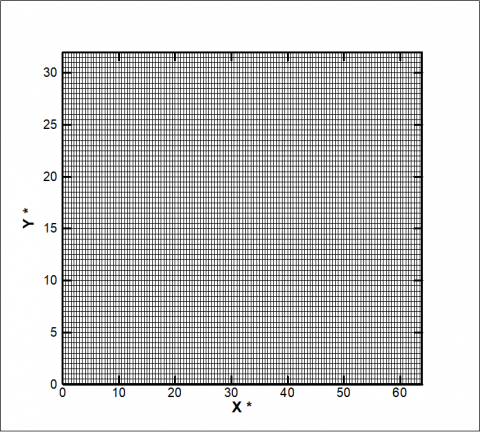Figure 3. Rectangular uniform structured mesh in two dimensional

Air is considered as a working fluid and ideal gas in both mixing layers. Since in the compressible flow the energy equation is coupled with the momentum equation, ideal gas law should be applied to solve these equations. The thermal conductivity and specific heat capacity at constant pressure are assumed to be constant and equal to 1006.43(j/kg) and 0.0242(w/mk), respectively. The specific heat ratio for air is equal to 1.4. Sound speed in air as an ideal gas is calculated from:

$a=\sqrt{\gamma R T}$    (56)

where R for air is equal to 287(j kg/k). Since the flow is supersonic and high speed, viscosity is determined through Sutherland's’ law based on three coefficients ($\mathrm{S}, \mathrm{T}_{0}, \mu_{0}$). Sutherland's’ law is written as:

$\mu=\mu_{0}\left(\frac{\mathrm{T}}{\mathrm{T}_{\mathrm{o}}}\right)^{3 / 2} \frac{\mathrm{T}_{0}+\mathrm{S}}{\mathrm{T}+\mathrm{S}}$    (57)

where, T is temperature, μ is viscosity, T0 is reference temperature and μ0 is reference viscosity. μ0 and T0 for the air are 1.716e-05(kg m/s), 273.11(k), respectively. S is a constant used in Sutherland's’ law which is 110.56 for the air.

Simulation details in convective Mach number of 0.64 shown in Table 1. Subscripts 1 and 2 in the following table are indicating characteristics of upper and lower speed of flows, respectively.

Table 1. Simulation details in $M_{c}=0.64$

 air-air Composition 1041.57,651.62 U1,U2 (m/s) 347.19,262.75 a1,a2(m/s) 0.62 r=U2/U1 3,2.48 M1,M2 0.64 Mc 101325,101325 P1,P2(pa) 1 P2/P1 1.177,2.06 ρ1,ρ2(kg/m3) 1.75 S= ρ2/ρ1 0.57 T2/T1 300,171.43 T1,T2(K) 1,1 δ1,δ2(cm) 0.16×104 Reynolds

Vorticity thickness is one of the significant parameters in analyzing of mixing layers which describes how the layers are mixed, described as:

$\delta_{\omega}=\frac{\Delta \mathrm{U}}{\left(\frac{\partial \mathrm{u}}{\partial \mathrm{y}}\right) \max }$    (58)

## 4.pngFigure 4. Variation of vorticity thickness along the x-axis

where, $\Delta \mathrm{U}$ and $\delta_{\omega}$ indicate velocity difference of upper and lower speed of flows and vorticity thickness, respectively. Fig.4 illustrates the variations of vorticity thickness versus spatial coordinate along the streamwise direction (x). Fig.4 indicates that with increase in x, vorticity thickness increases linearly to x=0.15 and then increases nonlinearly. The increase of vorticity thickness along the x-axis is due to the decrease of slope in velocity profiles. The results of the present simulation are compared with the results of LES obtained by Doris et al. . The results of Doris are obtained at the convective Mach number of 0.64 which is equal to the Mach number of present simulation. Fig. 4 demonstrates a good agreement between the results of present simulation and Doris. The result of vorticity thickness is done for two different meshes (128×64×64) and (192×96×96).

The velocity profiles are drawn in the xy plane. Fig. 5 shows the velocity profiles of mixing layers at different sections along streamwise direction. In addition, the velocity profiles of present simulation are compared with the velocity profiles obtained by Doris et al.  different sections of the flow. The border of two layers (Y*=16) is shown by dashed lines where is the starting place of mixing of layers. It is clear from velocity profiles, by approaching to the end of flow boundaries, the slope of velocity profiles in the mixing region decreases. The intersection point of border of two layers and velocity profiles at $x^{*}=60$ is an inflection point.

## 5.pngFigure 5. Velocity profiles

Another important parameter in order to analyze mixing layers is mixing layers growth rate (mixing rate) which indicates mixing characteristics and the compressibility effects which can be written as:

$\frac{d \delta}{\mathrm{dx}}=-\frac{4}{\left(U_{1}+U_{2}\right) \rho_{1} \Delta U^{2}} \int\left(\overline{\rho \mathbf{u}^{\prime \prime}_{1} \mathbf{u}^{\prime \prime}_{2}} \partial_{2} \tilde{\mathbf{u}}_{1}\right) \mathrm{d} \mathbf{y}$    (59)

In addition to the layers velocity, this parameter depends on layers density. For this purpose, Fig. 6 demonstrates the variations of growth rate of mixing layers versus convective Mach number. Mach number has significant role in simulation of mixing layers. Fig. 6 shows the comparison of the present simulation results with the experimental data obtained by Goebel & Dutton  and the results of DNS obtained by Pantano & Sarkar  for the variations of the growth rate versus the convective Mach number ranging from 0 to 1. In present simulation, the value of growth rate at convective Mach number of 0.64 is equal to 0.55 which is in good agreement with the experimental data and DNS results. The comparison of the results of growth rate for three cases is shown in Table 2. Another important point can be found from Fig. 6 and Table 2, is that the mixing layers growth rate should decrease when the convective Mach number increases which is consistent with the physics of problem.

Table 2. Comparison of mixing layers growth rate for three cases

 Growth Rate Mc NAME 0.55 0.64 Present Simulation 0.575 0.58 Goebel & Dutton 0.4 0.7 Pantano & Sarkar

## 6.png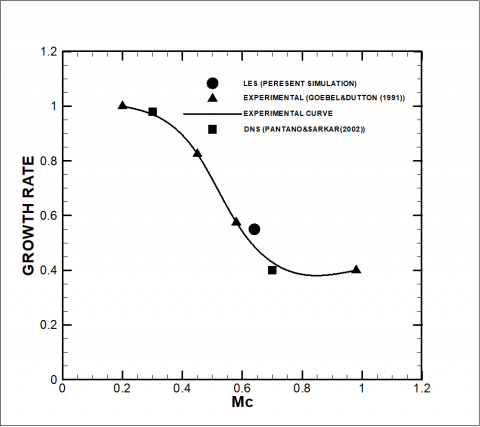Figure 6. Variation of growth rate versus $M_{c}$

In Fig. 7, the variation of Cd versus t* is shown. Dynamic coefficient is a fundamental parameter in modeling of turbulent stress tensor and $\alpha_{1}$ based on the dynamic eddy viscosity model. As it is clear from the Fig. 7, in order to prevent instability, Cd should be greater than and equal to zero. The dynamic coefficient indicates the flow turbulence as it is clear from Fig. 7, because of the turbulent nature of mixing layers, the flow turbulence occurs from the beginning of the flow. Fig. 7 illustrates the comparison of the values of this parameter in present simulation with the ones obtained by Verman  in temporal mixing layers at various times.

## 7.png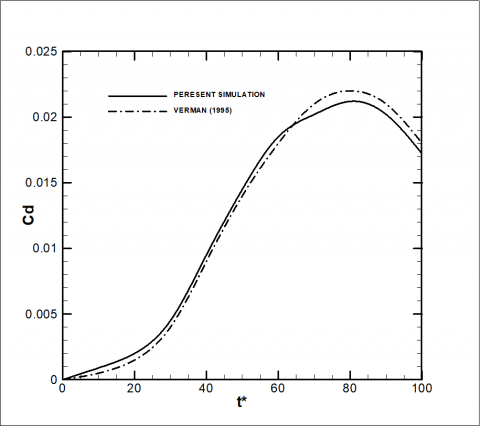Figure 7. Variation of Cd versus t*

The inverse of the Prandtl number plays a significant role in the modeling of $\alpha_{2}$ and $\alpha_{3}$ based on the dynamic eddy viscosity model. Fig. 8 illustrates the comparison of the values of this parameter in present simulation with the ones obtained by Verman  in temporal mixing layers at various times. The value of this parameter within $0<t^{*}<20$ is zero and therefore, turbulent stress tensor and $\alpha_{1}$ should be only modeled at this interval. As shown in Fig. 8, this parameter should be greater than and equal to zero.

## 8.pngFigure 8. Variation of $\frac{1}{\operatorname{Pr}_{t}}$ versus t*

## 9.png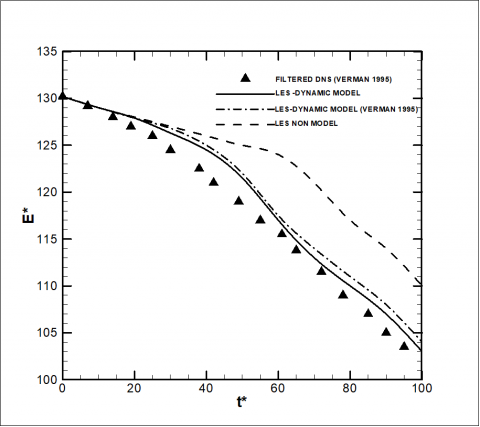Figure 9. Variation E* of versus t*

Fig. 9. shows the variation of non-dimensional total kinetic energy versus time. The total kinetic energy and the non-dimensional total kinetic energy are defined as:

$E=\int \frac{1}{2} \overline{\rho} \tilde{u}_{i} \tilde{u}_{i} d x$    (60)

$E^{*}=\frac{17.5 \mathrm{M}_{1} \mathrm{M}_{2} \mathrm{E}}{\mathrm{E}_{\mathrm{in}}}$    (61)

where, Ein indicates the initial kinetic energy. Fig.9. shows the comparison between the values of E* in this simulation using the dynamic eddy viscosity model and without sub-grid scales model with the results of dynamic eddy viscosity model and the results of filtered DNS obtained Verman . The dynamic eddy viscosity model of present simulation is in good agreement with filtered DNS but the non sub-grid scales model is not. From Fig.9, it is found that with the increase t*, the total kinetic energy decreases due to the transferred kinetic energy to heat by eddy viscosity in sub-grid scales. Fig.­ 9 shows that the total kinetic energy of E*=27.2 is dissipated within 0<t*<100 .

The most important parameter in qualitative analysis of mixing layers is vorticity. Fig. 10 and Fig.11 demonstrate vorticity color contour and contour lines, respectively. Fig. 12 shows the velocity vectors, which form the vortices in mixing layer. This contour shows how the mixing of layers and vortex formation across the flow field. The vorticity magnitude defined as:

$\omega=\sqrt{\omega_{\mathrm{x}}^{2}+\omega_{\mathrm{y}}^{2}+\omega_{\mathrm{z}}^{2}}$    (62)

## 10.pngFigure 10. Contour of vorticity in two dimensional domains

## 11.png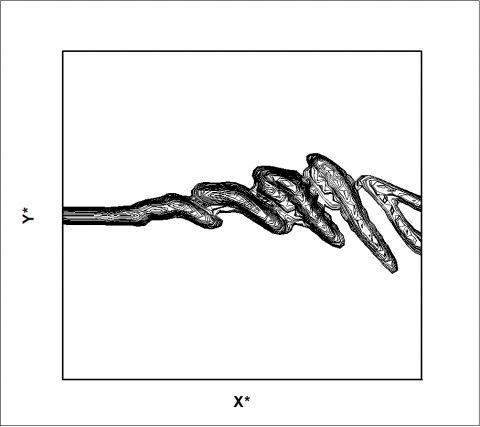Figure 11. Contour of vorticity in two dimensional domains

Fig. 10 shows that the vorticity form in the middle of the plane (at the border of two flows) and the mixing rate of layers and the values of vortices increase by approaching to the end of the flow. As seen from the Fig. 10, because of the turbulent nature of mixing layers, the flow become turbulent quickly and vortex and vortices form. The vorticity values should be maximized in the center of vortexes and as seen from Fig. 10, the vorticity magnitude increases by approaching to the center of vortexes. Fig. 11 indicates that vortices divided into two parts after entering to flow and then these two parts divided into many parts and this process is continued. The kinetic energy transfer from large to small eddy and from small to smaller eddy is the reason of eddy divisions. Finally, this energy is converted to heat by eddy viscosity. Fig. 12 shows the velocity vectors forming vortices. As it is clear from this Figure, the vortices form in the mixing region.

## 12.pngFigure 12. Contour of vorticity in two dimensional domains

## 13.png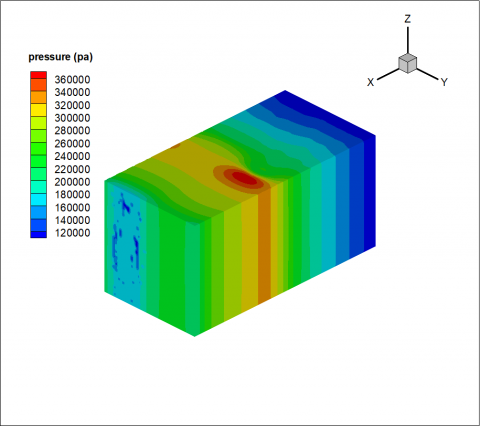Figure 13. Contour of pressure in three-dimensional domain

A three-dimensional pressure in the aspect of quality is shown in Fig. 13. Since the flow is supersonic, it is expected to have shock in the pressure contour. Shocks are the sudden changes of pressure and formation of severe pressure gradients in the flow. The shock occurs in the flow is investigated in Fig. 13. By approaching to the center of shock, Pressure increases and at the center of the shock the pressure reaches its maximum value while, it decreases far from the shock center. This shock occurs at 33<X*<45 and its center is at point of (39, 24, 16). The pressure lines forming the shock are shown clearly in Fig.14. Fig. 15 shows the pressure contour in the outlet plane. Two important points can be found from Fig.15. First, the shock is symmetric along the Z*=16. Secondly, group of pressure gradient occur at 2<Y*<15  in the outlet plane which are smaller than investigated shock. It is necessary to have the variation of pressure along the x axis in free slip flow (xy) in order to obtain the pressure gradient at the beginning of the shock occurrence to the center of shock. Fig. 16 indicates the non-dimensional pressure ($P^{*}=\frac{P}{P_{\text { in }}}$) versus x in the periodic and free slip wall planes where Pin is initial pressure. It is obtained from the figure that the value of pressure gradient at the beginning of shock occurrence to the center of shock is 0.45.

## 14.pngFigure 14. Contour of pressure in two-dimensional domain

## 15.pngFigure 15. Contour of pressure in two-dimensional domain

## 16.pngFigure 16. Variation P* of versus X*

7. Conclusion

In this article, the results of LES in the spatially developing compressible mixing layers in convective Mach number of 0.64 are presented. The mixing layer is a fundamental case for the large eddy simulation of compressible flows. The dynamic eddy viscosity model is applied for modeling of sub-grid scales. The cell-centered finite volume method with second order of accuracy is used for spatial discretization. The viscous and convection terms are discretized separately by second order schemes. The second order implicit method is used in order to temporal discretization. Present simulation results are good agreement in comparison with available experimental and DNS and other LES results in the same convective Mach number. In present simulation, the value of growth rate at convective Mach number of 0.64 was equal to 0.55. The decrease of growth rate with the increase of convective Mach number in applied simulation is predicted. The total kinetic energy decreases due to the transferred kinetic energy to heat by eddy viscosity in sub-grid scales. The total kinetic energy of 27.2 is dissipated of the flow field. Finally, the shock occurs in the flow are investigated. This shock occurs at 33<X*<45 and its center is at point of (39, 24, 16). The value of pressure gradient at the beginning of shock occurrence to the center of shock is 0.45.

References

 Germano M, Piomelli U, Moin P, Cabot WH. (1991). A dynamic sub-grid scale eddy viscosity model. Phys. Fluids A. 3(7): 1760-1765.

 Smagorinsky J. (1963). General circulation experiments with the primitive equations. Mon. Weather Revue 91: 99-164.

 Bognadoff DW. (1983). Compressibility effects in turbulent shear layers. AIAA J. 21: 926-927.

 Doris L, Tenaud C, Phuoc LT. (2000). LES of spatially developing 3d compressible mixing layer. C. R. Acad. Sci. Paris Manqué des fluids numrique. t. 328 (Srie II b) 567–573.

 Bruin ID. (2001). Direct and Large Eddy Simulation of the Spatial Turbulent Mixing Layer. PhD thesis. Twente University Press 79-105.

 Jones WP, Lyra S, Marquis AJ. (2009). Large eddy simulation of a droplet laden turbulent mixing layer. International Journal of Heat and Fluid Flow 31: 93-100.

 Goebel SG, Dutton JC. (1991). Velocity measurements of compressible turbulent mixing layers. AIAA J. 29(4): 538-546.

 Verman AW. (1995). Direct and Large Eddy Simulation of the Compressible Turbulent Mixing Layers. PhD Thesis. University of Twente 1-150.

 Pantano C, Sarkar S. (2002). A study of compressibility effects in the high speed turbulent shear layer using direct simulation. J. Fluid Mech. 329-371.

 Kourta A, Sauvage R. (2002). Computation of supersonic mixing layers. Physics of Fluids 14(11): 3790-3797.

 Freud JB, Lele SK, Moin P. (2002). Compressibility effects in a turbulent annular mixing layer. J. Fluid Mech. 421: 229-267.